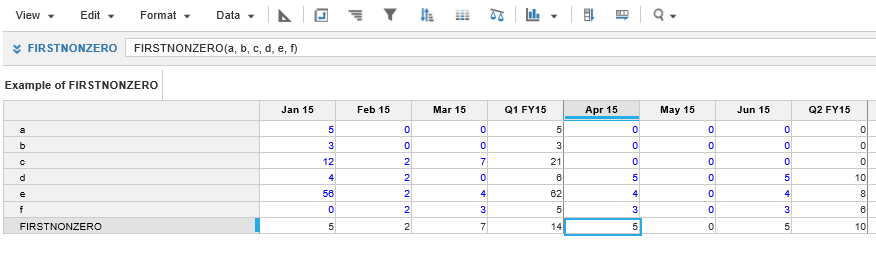1. Calculation functions
2. All Functions
3. Numeric Functions
4. FIRSTNONZEROReturns the first of at least two arguments that is non-zero.

This function can be used to avoid a complex conditional:

`FIRSTNONZERO(a, b, c)    `

`IF a <> 0 THEN a ELSE IF b <> 0 THEN b ELSE IF c <> 0 THEN c ELSE 0.`

If any argument is NaN this will also be skipped, but Infinity and -Infinity are treated as non-zero values. These non-numeric values do not occur often, but can crop up as the result of certain calculations e.g. DIVIDE(1, 0), DIVIDE(0, 0), DIVIDE(-1, 0).

## Syntax

`FIRSTNONZERO(a, b, c) `

where:

• a: Number
• b: Number
• c: Number

## Format

Input Format Output Format
a, b, c : Number Number

## Arguments

The function uses the following arguments:

• a, b, c: Number: Numeric line item, property, or expression

## Constraints

The function has the following constraints:

• Function only accepts numbers

## Excel equivalent

• No Excel equivalent

## Example

In the example shown, the first six rows hold the data for each month. The last row holds the formula

`FIRSTNONZERO(a,b,c,d,e,f)`

that shows the first non zero value each month.